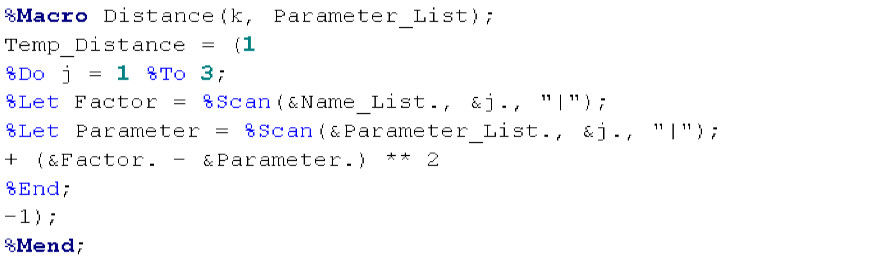top of page
Search

# K-Means In BASE SAS

Following on from last week’s well received post on PCA in Base SAS this week I am going to use Base SAS for K-Means. K-Means is a way of making predictions by assessing how much a new observation is like existing observations. It works in a similar way to grid references on a map. So we know that Florence is similar to Pisa and Cardiff is similar to Newport but Florence is not very similar to Newport. Although there are a host of reasons for this, proximity is a good proxy for these historical and cultural differences.

K-Means usually relies on calculating the Euclidean distance between points in a multi-dimensional space. This is computationally very demanding. It requires making many calculations which can be time consuming. Thankfully one of the advantages of SAS is its speed at exactly this kind of problem.

So I have taken a set of data on electricity consumption by each postcode in the UK downloaded from www.gov.uk. I am going to use a few simple attributes about each of these postcodes to try to predict the electricity consumption. The input factors are the longitude and latitude of the centre of each postcode and the number of electricity meters in each postcode. These are from the Office of National Statistics.

First I split the data into two sets using a random number seeded with today’s date. The first one will be used to build a k-means model and the second will be used to test the model.Second I will use the SAS procedure FastClus on the first set of data. This is a clustering procedure which divides up the sample space into a given number of clusters. The cluster centers are chosen by minimizing the sum of squares distance from each observation to the center of its nearest cluster. I have chosen to use 3,000 clusters in this example but I have set this as a macro variable so the code could be re-run with a different number of clusters. The outputs from this procedure are the original dataset but with the number of the cluster to which each point belongs and a dataset with statistical information about each cluster.Thirdly I want the mean electricity consumption for each cluster and also a dataset with just the location of the center of each cluster. This needs to be transposed so it can be made into a macro variable.Thirdly I want the mean electricity consumption for each cluster and also a dataset with just the location of the center of each cluster. This needs to be transposed so it can be made into a macro variable.Next I will load each of the cluster centers into SAS as macro variables so I can test the second dataset.Now I am going to go through each observation in the second dataset and test which cluster center is its nearest. I do this by obtaining the distance to the first cluster center and then seeing if the second cluster center is closer. I then repeat this for all of the clusters. I only store the distance to the current closest cluster to reduce the amount of stored data.This is by far not the most efficient way to do this step. But it is a very simple way and actually runs in a very reasonable time of about three minutes. On larger datasets with more dimensions there are several algorithms that can be implemented to speed this step up.

So finally I will test how accurate are the predictions of electricity consumption.So that is a roughly six step process for using Base SAS for K-Means. In this example the model predicts 27% of postcodes to within 10% of their actual electricity use. The gini co-efficient is 0.33. So not bad but not great either. This could be easily improved by adding in additional factors.

Incidentally I often find it useful to suppress the SAS log when performing multiple repeated macro tasks using the below line of code. This seems to add an extra bit of speed.Below is the code as text. Thanks for reading.

Data Sample_1 Sample_2;

Set Electricity_Consumption_PostCode;

If uniform(today()) <= 0.5 Then Output Sample_1;

Else Output Sample_2;

Run;

%Let ClusterCount = 3000;

Proc Fastclus Data=Sample_1 Out=Sample_1_Clusters

Maxclusters=&ClusterCount.

Outstat=ClusterCentres;

Var Longitude Latitude Meter_Count;

Run;

Proc Summary Data=Sample_1_Clusters nway missing;

Class Cluster;

Var Consumption;

Output Out=MeanTable

(Rename = (Consumption = P_Consumption))

Mean=;

Run;

Data ClusterCentres(Drop=_TYPE_ OVER_ALL CLUSTER Consumption);

Set ClusterCentres(Where=(_TYPE_= "CENTER"));

Run;

Proc Transpose Data=ClusterCentres Out=Temp_ClusterCentres; Run;

Proc sql noprint;

Select _NAME_

Into :Name_List separated by '|'

From Temp_ClusterCentres;

Quit;

%Do j = 1 %To &ClusterCount.;

%Global Parameter_List_&j.;

Proc sql noprint;

Select Col&j.

Into :Parameter_List_&j. separated by '|'

From Temp_ClusterCentres;

Quit;

%End;

%Mend;

%Macro Distance(k, Parameter_List);

Temp_Distance = (1

%Do j = 1 %To 3;

%Let Factor = %Scan(&Name_List., &j., "|");

%Let Parameter = %Scan(&Parameter_List., &j., "|");

+ (&Factor. - &Parameter.) ** 2

%End;

-1);

%Mend;

%Macro CalculateDistance();

Data Sample_2_Testing;

Set Sample_2;

%Distance(1, &Parameter_List_1);

Cluster = 1;

Distance = Temp_Distance;

Run;

%Do k = 2 %To &ClusterCount.;

Data Sample_2_Testing(Drop=Temp_Distance);

Set Sample_2_Testing;

%Distance(&k., &Parameter_List_&k.);

If Temp_Distance < Distance Then Do;

Distance = Temp_Distance;

Cluster = &k.;

End;

Run;

%End;

%Mend;

%CalculateDistance();

Proc Sql noprint;

Create Table Merged as

Select a.*, b.P_Consumption

From Sample_2_Testing a

Left Join MeanTable b

On a.Cluster = b.Cluster;

Quit;

Data Merged; Set Merged;

Within_010Percent = (abs(P_Consumption / Consumption- 1) < 0.1);

Run;

Proc Freq data=Merged;

Table Within_010Percent;

Run;

1 view

See All
bottom of page